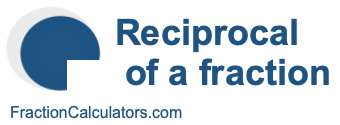Reciprocal of 5/10What is the reciprocal of 5/10? Here we will define reciprocal of 5/10, and show you how to calculate the reciprocal of 5/10 in fraction form and decimal form.

The reciprocal of 5/10 is a fraction or a number that when multiplied by 5/10 is equal to 1. To get the reciprocal of 5/10 based on that definition, we can make the following equation where "R" is the reciprocal of 5/10.

(5/10) × R = 1

When we solve for R, we get the answer to the reciprocal of 5/10 as a fraction as follows:

(5/10) × R = 1
R = 10/5
Reciprocal of 5/10 = 10/5

You can check that the answer is correct by confirming that 5/10 times the reciprocal of 5/10 is equal to 1, like this:

5/10 × 10/5 = 1

To find the decimal answer to the reciprocal of 5/10, you divide the numerator of the reciprocal by the demoninator of the reciprocal, like this:

10 ÷ 5 = 2
Reciprocal of 5/10 = 2

Tip: As you may have inferred from our tutorial above, you can quickly get the reciprocal of any fraction, such as 5/10, by switching the numerator and the denominator.

Reciprocal of a fraction
Do you need the reciprocal of another fraction? No problem! Please enter another fraction below.

Reciprocal of /

Reciprocal of 5/11
Here is the next fraction on our list that we have calculated the reciprocal of.